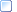﻿ The FORCE_PLATFORM group

## The FORCE_PLATFORM group

The FORCE_PLATFORM group is used to store information about the type, location, orientation, and implementation of the force plates within the 3D data collection environment.  Force plates are used to measure applied forces and moments – typically the ground reaction forces and moments produced by human gait although other applications exist, for example running, rock climbing, pole vaulting, cutting maneuvers, and other agility exercises that generate unique forces and moments.

The FORCE_PLATFORM group must be present whenever a C3D file contains analog data from force platforms because it describes the type of force platforms used, their locations within the calibrated 3D data recording volume, the assignment of force plate signals to specific analog channels, as well as documenting the force plate information required to calibrate and interpret the data generated by the force platform.  This is one of the more complex parameter groups to set up but it is usually only done once for each data collection environment and once it has been configured it need not be changed unless the force plates change their location within the calibrated 3D data collection volume.

Since applications use the parameters from this group to process the force plate data in the C3D file the FORCE_PLATFORM:USED parameter must be set to zero if no valid force plate data is present.  This enables applications to determine that the C3D file does not contain any force platform data and ignore all other FORCE_PLATFORM group parameters while preserving the data collection environment configuration.

The C3D file format records force platform data as analog values stored in analog channels, each with an associated analog scaling factor that translates the stored data values into forces and moments.  There is no requirement that force platform data stored in any specific order as the FORCE_PLATFORM:CHANNEL parameter is used to specify the correspondence between recorded analog data channels (1, 2, and 3 etc.) and force platform channels (e.g.  Fx, Fy, Mz).  It is recommended that the analog data channels are assigned force platform data in the same order in each recording environment if possible.

The physical location and orientation of the force platform within the 3D data collection space is defined by the FORCE_PLATFORM:CORNERS parameter.  This parameter defines the location of the corners of the platform in 3D space and the order in which the corners are specified provides the rotational alignment between the 3D coordinate system and the force plate coordinate system, allowing the computation of force vectors, center of pressure, etc., in the calibrated 3D space.

Analog data from the force platform is scaled using the ANALOG:GEN_SCALE, ANALOG:SCALE, and ANALOG:OFFSET parameters that are applied to the analog data before its use in the force plate computations.  The analog data is stored within the C3D file within the range of the range of the recording hardware (the ADC card) e.g., -5 volts to +5 volts, or -10 volts to +10 volts.  The ANALOG:OFFSET, SCALE and GEN_SCALE factors are used to convert the recorded analog data to force and moment values while the OFFSET is simply the data value corresponding to 0 volts of input.  The ANALOG:OFFSET value is subtracted from each analog data value before the scale factor for the channel is applied.

Two values must be determined before it is possible to calculate the scale factors for each force plate channel.  These are the force plate sensitivity value, and the ADC sensitivity value:

 Each individual force plate output channel has a value associated with it by the manufacturer that expresses the sensitivity of the channel – generally in terms of the amount of force required to produce one volt of output or the moment that must be applied to the plate to produce one volt of output.  This information is usually available from the manual supplied by the force plate manufacturer.

 The ADC sensitivity value is expressed in units of volts per bit, where a bit is a raw analog data unit (4095 bits will correspond to full scale for a 12-bit ADC).  The ADC sensitivity depends on both the hardware range setting of the ADC as well as any gains that are applied to the signal, in either hardware or software before the data is recorded.

If the force plate sensitivity for a given channel is S, and the ADC sensitivity is A, then the value to enter into the ANALOG:SCALE parameter for that channel is A*S, i.e., the units for the scale factor parameter must be force/bit or moment/bit.  If the parameter ANALOG:GEN_SCALE is not set to 1.0, then the value of A must be first divided by the value used in ANALOG:GEN_SCALE.

Alternately, the ANALOG:GEN_SCALE parameter may be set to the value of A, then the ANALOG:SCALE factors can be set to the values of S for each individual channel to provide the desired result.  Care must be taken to use consistent units i.e., if force is being expressed in newtons, the moments should be in newton-millimeters (Nmm) or newton-meters (Nm).

A full discussion of all the factors involved in calculating analog scaling factors can be found in the discussion of the ANALOG:SCALE parameters – refer to this for complete details (including worked examples) of the calculations.

More:Specifying Force Platform ParametersFORCE_PLATFORM:USEDFORCE_PLATFORM:TYPEFORCE_PLATFORM:ZEROFORCE_PLATFORM:CORNERSFORCE_PLATFORM:ORIGINFORCE_PLATFORM:CHANNEL